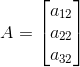Let A be a matrix of order 3 × 4. If R1 denotes the first row of A and C2 denotes its second column, then determine the orders of matrices R1 and C2.

Asked by Aaryan | 1 year ago |  91

##### Solution :-

Given A be a matrix of order 3 × 4.

So, A = [ai j3×4

R1 = first row of A = [a11, a12, a13, a14]

So, order of matrix R1 = 1 × 4

C2 = second column ofTherefore order of C2 = 3 × 1

Answered by Sakshi | 1 year ago

### Related Questions

#### Find the values of a, b, c and d from the equations

Find the values of a, b, c and d from the equations:

#### Find x, y, a and b if

Find x, y, a and b if

#### Find x, y, a and b if

Find x, y, a and b if

Construct a 4 × 3 matrix A = [ai j] whose elements ai j are given by $$a_{i j} = \dfrac{(i – j)}{(i + j)}$$